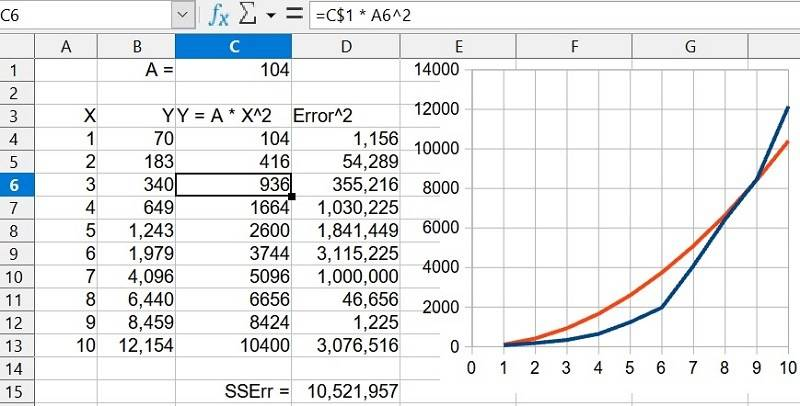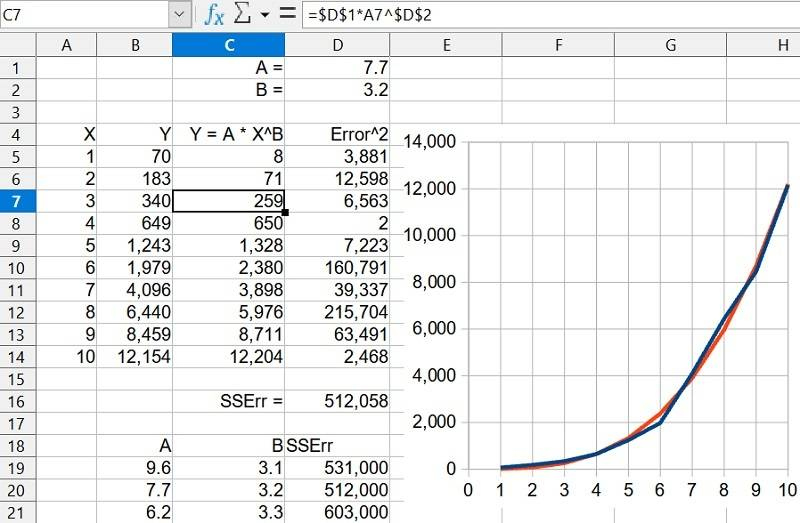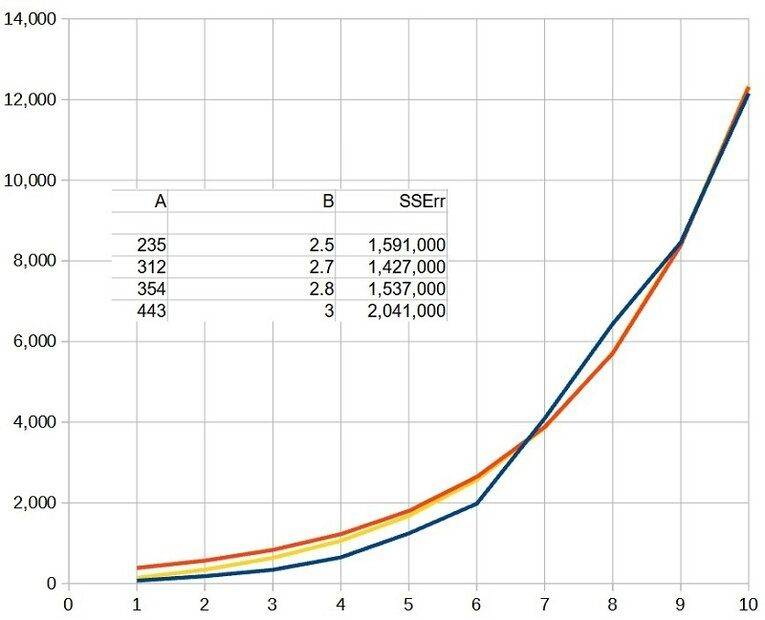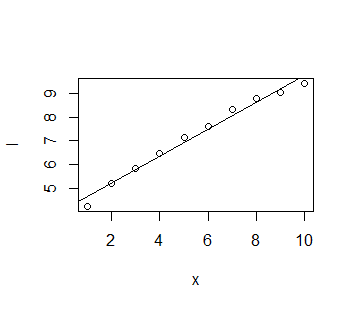# Is this business graph an exponential or polynomial function?

Summary:
exponential or polynomial function
GRAPH WITH VALUES:
Sorry I have a small dilema, I don't know if this is a exponential or polynomial function. I'd think its exponential but it doesn't have same change of factors.

fresh_42
Mentor
It looks exponential, but with only finitely many points given, you can always find a polynomial through those points. Such a polynomial would be of degree nine in your case.

•docnet and jorgevf200002
docnet
Gold Member
It looks exponential, but with only finitely many points given, you can always find a polynomial through those points. Such a polynomial would be of degree nine in your case.
May I ask how in the world you knew the polynomial would be of degree nine?

fresh_42
Mentor
May I ask how in the world you knew the polynomial would be of degree nine?
We have ten points ##(x_0,y_0)##. Each gives a linear equation ##y_0=a_0+a_1x_0+a_2x_0^2+\ldots+a_nx_0^n##. Thus I have ##10## linear equations in the unknowns ##a_0,a_1,\ldots,a_n##. With ##n=9## we get ##10## equations and ##10## variables in a linear system. The chances that the coefficient matrix is not regular are practically zero.

•docnet
Mark44
Mentor
To elaborate on what @fresh_42 said, if you have two points, a linear polynomial (degree 1) can be fitted; with three points, a quadratic polynomial (degree 2) can be fitted. In general, if there are N points, a polynomial of degree N - 1 can be fitted.

•docnet
Svein
A standard way of testing if the graph is more or less exponential:
1. Take the logs of all the values
2. Find the best least-squares line through the logs
3. (Check the "goodness of fit", r2)
4. Calculate a new set of values from the least-square formula
5. Take exponentials of the new values
6. Plot the originals and the calculated new values in the same graph
7. See if it looks promising
Remember: All measured data contain noise - even if you suspect a perfect fit, you will not get it.

••DaveE, hutchphd, berkeman and 2 others
Office_Shredder
Staff Emeritus
Gold Member
The data is obviously a little noisy - we wouldn't require an exponential curve to literally pass through all the points, so we shouldn't require a polynomial to do so either. A degree four or five polynomial might fit it really well.

That said, you almost certainly want the exponential fit. The polynomial you fit is going to do pretty random stuff to the left and right of your data, the exponential will keep growing in an increasingly steep curve which is probably correct. Since the graph is in excel, I think you can just get it to graph best fit polynomials and exponentials for you to see what they look like.

That said, the slope looks like it might hit zero at 0, so maybe this is really just a quadratic polynomial (which should have better extrapolation properties).

•DaveE
berkeman
Mentor
Summary:: exponential or polynomial function

GRAPH WITH VALUES:
Sorry I have a small dilema, I don't know if this is a exponential or polynomial function. I'd think its exponential but it doesn't have same change of factors.
BTW @jorgevf200002 -- Is this a schoolwork question? Your "Sales vs. Year" graph looks like it's from a course, not from your business...

•docnet
fresh_42
Mentor
BTW @jorgevf200002 -- Is this a schoolwork question? Your "Sales vs. Year" graph looks like it's from a course, not from your business...
I think neither. My guess is, that it is either a wet dream or a business plan for the bankers.

•SammyS and berkeman
Exponential growth is the standard model for this sort of stuff, have never seen a polynomial sales projection

•berkeman
OmCheeto
Gold Member
I'm not really sure why it can't be both, as when I find the log values as recommended by Svein in post #6, I get a curve.
The R2 values are interesting in that it appears to be better fit by a quadratic curve than a system generated exponential curve.

Last edited:
jrmichler
Mentor
When curve fitting, I normally use a spreadsheet to find the mathematical curve. Excel has a good solver, but LibreOffice does not, so I use a manual gradient search to minimize the sum squared error. I also like to plot the mathematical curve on top of the experimental data. An example quadratic fit for the data in the OP:I next tried an equation of the form ##Y = A*X^n##, where both A and n are variables. This one has two variables, so it took about ten minutes to iterate both A and n. The optimal approach is to choose a value for n, then iterate A for minimum sum square error (SSErr in spreadsheet). Choose a new n, repeat. The results:The table in rows 18 to 21 shows the effect of iterating the exponent. It would be easy to get another decimal place of accuracy in the exponent, but the resulting decrease in error would be minimal. I also see no need to add additional terms to this equation.

The same approach is easily adaptable to exponential, trigonometric, or other forms. Although more than two variables can be challenging to curve fit by manual methods. I like this method because I have direct visual evidence of the quality of the curve fit, it can be done with any spreadsheet, and it's easy.

•OmCheeto and berkeman
However this is a ‘business graph’ so if the number is, say, revenue you trivially know what the function is - sum(all sales in the business for the year). Sales then is a result of all the business operations - marketing, ops etc. Its trivial, but important to remember the reality behind the numbers and how crude an approximation will be by any simple equation

given that sales, or any other metric measuring growth in dollars is going to be heteroskedastic, a good first step is to take differences, maybe regressing on the log change - you then have linearized the data and OLS works fine. You can then add other explanatory variables if needed

Logically, it has to be some sort of logistic function - exponential in the early growth stages then flattening out as the business matures

Last edited:
FactChecker
Gold Member
The rate of growth increases like an exponential function. You can graph the logarithm of the Y values. If they lie on a straight line, then the original data are exponential.

jrmichler
Mentor
I tried an exponential function on the data in the OP, ##Y = Ae^{X/B}##. That did not fit well, so I subtracted the A value: ##Y = -A + Ae^{X/B}## to force the equation to start at 0, 0. The optimized results of both of these equations are plotted below.Summarizing the equations and sum of square errors for the above curve fits:
Equation ................ SSError
##Y = X^2## ... ....... 10,522,000
## Y = AX^n## ... .......... 512,000
##Y = Ae^{X/B}##......... 2,191,000
##Y = -A + Ae^{X/B}##... 1,427,000

It looks like the polynomial of degree 3.2 is by far the best fit.

But simple curve fitting is (usually) not the best way to fit curves to data. The best way is to start by looking for the math underlying the original data. If the original data is from population growth with exponential basis, then the curve fit should be exponential, even if a polynomial would better fit the data.

Last edited:
•OmCheeto
Chestermiller
Mentor
Have Excel plot the data using a log scale for the ordinate and see what you get.

•FactChecker
FactChecker
Gold Member
Have Excel plot the data using a log scale for the ordinate and see what you get.
I agree. That would be my first recommendation.
Here is what I got from an R program:Residuals:
Min 1Q Median 3Q Max
-0.39081 -0.11329 0.07758 0.14110 0.26229

Coefficients:
Estimate Std. Error t value
(Intercept) 4.06994 0.16508 24.65
x 0.56936 0.02661 21.40
Pr(>|t|)
(Intercept) 7.83e-09 ***
x 2.39e-08 ***
---
Signif. codes:
0 ‘***’ 0.001 ‘**’ 0.01 ‘*’ 0.05 ‘.’ 0.1 ‘ ’ 1

Residual standard error: 0.2417 on 8 degrees of freedom
Multiple R-squared: 0.9828, Adjusted R-squared: 0.9807
F-statistic: 458 on 1 and 8 DF, p-value: 2.392e-08

Chestermiller
Mentor
I agree. That would be my first recommendation.
Here is what I got from an R program:
View attachment 288734

Residuals:
Min 1Q Median 3Q Max
-0.39081 -0.11329 0.07758 0.14110 0.26229

Coefficients:
Estimate Std. Error t value
(Intercept) 4.06994 0.16508 24.65
x 0.56936 0.02661 21.40
Pr(>|t|)
(Intercept) 7.83e-09 ***
x 2.39e-08 ***
---
Signif. codes:
0 ‘***’ 0.001 ‘**’ 0.01 ‘*’ 0.05 ‘.’ 0.1 ‘ ’ 1

Residual standard error: 0.2417 on 8 degrees of freedom
Multiple R-squared: 0.9828, Adjusted R-squared: 0.9807
F-statistic: 458 on 1 and 8 DF, p-value: 2.392e-08
Excel will do it automatically for you. All that needs to be done is to switch the vertical axis to a logarithmic scale with a single click.

•FactChecker